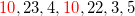# Algebra 1 : How to find mode

## Example Questions

### Example Question #21 : How To Find Mode

What is the mode?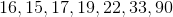NoneNone

Explanation:

Mode is the data point that occurs most frequently.

Let's arrange in increasing order.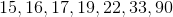.

All the numbers occur once so the answer is none.

### Example Question #22 : How To Find Mode

What is the mode?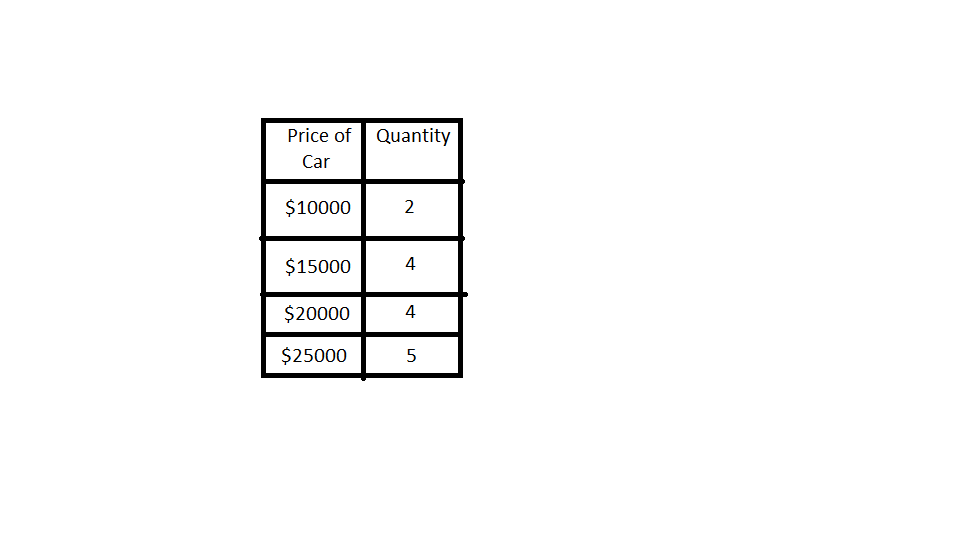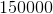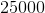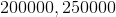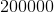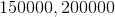Explanation:

Mode is the data point that occurs most frequently.

Based on this data,occurs the most at five times.

### Example Question #21 : How To Find Mode

What is the mode?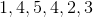Explanation:

Mode is the data point that occurs most frequently.

Let's arrange in increasing order. We have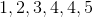occurs twice and is most frequent.

Therefore,is the correct answer.

### Example Question #24 : How To Find Mode

What is the mode?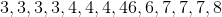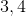Explanation:

Mode is the data point that occurs most frequently. Since it's in order, we look for the most frequent.

Three appears four times, four appears three times, 46, 6, and 8 appear once, and seven appears three times.

Therefore the mode is### Example Question #22 : How To Find Mode

Determine the mode: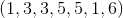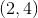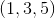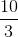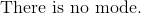Explanation:

Reorder the data set in chronological order.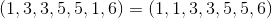The mode is the most frequent number that appears in the set.  There can be more than one mode in a set.  The most common numbers are 1,3, and 5.

The correct answer is:### Example Question #26 : How To Find Mode

Determine the mode of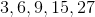.There is no mode for this setThere is no mode for this set

Explanation:

The mode is the number in a set that appears the most often. Each number in the given series is only seen once. Therefore, the solution is that there is no mode for this sereis.

### Example Question #27 : How To Find Mode

What is the mode of the numbered set?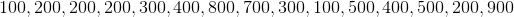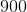The series has no mode.Explanation:

The mode is the most commonly displayed number in the data set. Unlike the median, this is not based on position within the set, so no reordering is (strictly speaking) required. The number 200 occurs four times in the set, with no other number occurring this often. Therefore, the set is unimodal (has one mode), and the mode is 200.

### Example Question #22 : How To Find Mode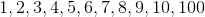Using the data above, find the mode.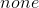Explanation:

The mode is the data value that appears the most often. In this set of data, each value appears only once, we have no numbers that are repeated. Thus, this data set has no mode, answer "none."

### Example Question #29 : How To Find Mode

Which of the following statements is true of this data set?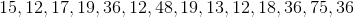The mode of the set is 12.

The set has more than one mode.

The set is bimodal.

The mode of the set is 36.

All of these statements are true.

All of these statements are true.

Explanation:

Mode refers to the value in a data set that occurs most often in the set. We can see when we rearrange the set: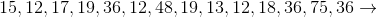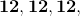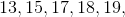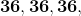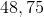That there are two values occurring three times each. Both are the mode, thus the system is bimodal.

### Example Question #23 : How To Find Mode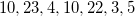Using the data above, find the mode.andThe mode is the piece of data that appears the most. In the set provided, each data piece appears once, all but the numberwhich appears twice. If this data were graphed on a histogram, the mode would be easily visualized as the bar, line, or point that reachest the highest value on the y-axis.